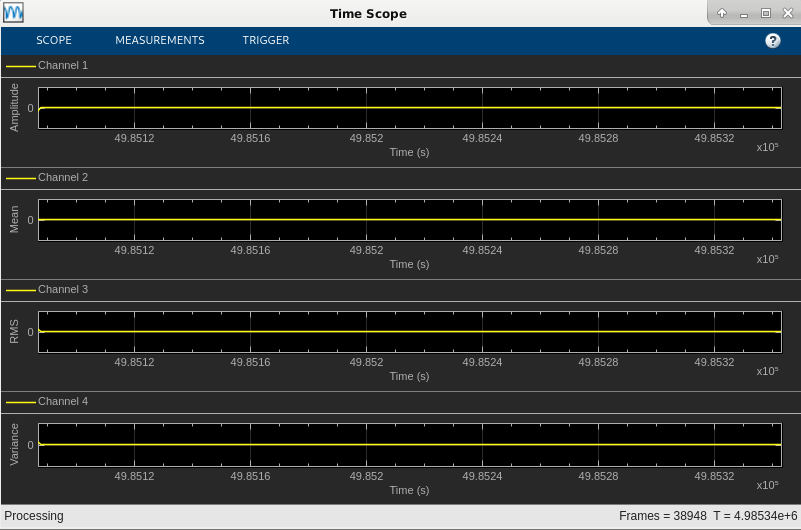# Generate Standalone Executable and Interact with It Using UDP

This example shows how to generate a standalone executable for streaming statistics using MATLAB® Coder™ and tune the generated executable using a user interface (UI) that is running in MATLAB®.

### Introduction

Most algorithms in DSP System Toolbox™ support C code generation using MATLAB Coder.

One of the options of MATLAB Coder is to generate a standalone executable that can be run outside of the MATLAB environment by launching the executable directly from a terminal or command prompt.

For algorithms that are tunable, it is desirable to interact with the algorithm at run-time using a UI. One way to achieve this is by sending/receiving information via UDP.

This example uses UDP to exchange between MATLAB and a generated standalone executable at run-time. The variance, bias, and exponential weighting values are sent from MATLAB to the executable. The actual random signal along with mean, RMS, and variance estimates are sent from the standalone executable back to MATLAB for plotting.

### Example Architecture

The architecture of the example consists of two primary sections:

1. `streamingStatsCodegenExampleApp`: A MATLAB function that creates the user interface (UI) to change the variance, bias, and exponential weighting values. This function also plots the data received from the standalone executable.

2. `HelperStreamingStatsEXEProcessing`: This is the function from which the standalone executable is generated. This function generates a random signal of a given bias and variance and computes mean, RMS, and variance estimates of such a signal. The noise signal along with the statistics that are computed are sent over UDP for plotting (or any further processing). Anytime during the simulation, it can also respond to the changes in the sliders of the MATLAB UI.

### Generating Code and Building an Executable File

You can use MATLAB Coder to generate readable and standalone C-code from the streaming statistics algorithm code. Because UDP is used, there are additional dependencies for the generated code and executable file. These are available in the /bin directory of your MATLAB installation.

Running the script `HelperStreamingStatsGenerateEXE` will invoke MATLAB Coder to automatically generate C-code and a standalone executable from the algorithm code present in `HelperStreamingStatsEXEProcessing`.

### Running the Example

Once you have generated the executable, run the function `streamingStatsCodegenExampleApp` to launch the executable and a user interface (UI) designed to interact with the simulation. The UI allows you to tune parameters and the results are reflected in the simulation instantly. For example, moving the slider for the 'Variance' while the simulation is running, will affect the noise signal along with the RMS and variance estimates that are plotted.There are also two buttons on the UI - the 'Pause Simulation' button will hold the simulation until you press on it again. The simulation may be terminated by clicking on the 'Stop simulation' button.In lieu of using the `system` command to launch the executable from within MATLAB, the executable can be launched manually from a terminal or command prompt. Because this executable includes UDP calls, it is necessary that the dlls be on the path for proper behavior. See How To Run a Generated Executable Outside MATLAB for more information.